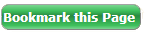SGXNIFTY DOWFUTURES LIVE
Pivot Point & Fibonacci Calculator

Pivot Point Calculator

The Pivot Calculator is powered by Investing.com

Fibonacci Calculator

The Fibonacci Calculator is powered by Investing.comLive World Indices are powered by Investing.com